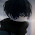Friction

MCQsQ1:  Which friction of the following is the highest?

(a) Sliding friction.
(b) Rolling friction
(c) Static friction
(d) None of these

Q2:  Friction is a ______.

(a) type of contact force.
(b) type of non-contact force.
(c) type of pressure.
(d) form of heat.

Q3: An object is at rest on a floor, a force is applied to move that object. Which friction force will come into an action at this moment?

(a) Sliding Friction
(b) Static Friction
(c) Rolling Friction
(d) Fluid Friction

Q4: Friction is a ....

(a) scalar quantity
(b) vector quantity
(c) neither (a) and (b)
(d) can be scalar or vector

Q5: A block is sliding on a horizontal surface. The friction can be increased by ...

(a) decreasing the mass of the block.
(b) decreasing the area of contact with surface.
(c) by polishing the surface.
(d) by putting second block on the top.

Q6: Friction always acts __________ the direction of motion of an object.

(a) in the same direction as
(b) perpendicular to
(c) opposite to
(d) at 45 degrees angle to

Q7: On which of the following toy car will travel the farthest?

(a) Wet marble floor
(b) Dry marble floor
(c) Newspaper
(d) Carpet

Q8: Rougher Surfaces have ...

(a) no friction
(b) less friction
(c) higher friction
(d) same level of friction.

Q9: Which of the following statement is NOT true?

(a) Friction make the things slow down.
(b) Friction produces heat.
(c) Friction can stop the moving object.
(d) Friction is not useful.

Q10: The force which opposes the movement of an object when it travels through air or water?

(a) Tension Force
(b) Drag Force
(c) Spring Force
(d) Gravitational force

1: (c) Static friction
2: (a) type of contact force.
3: (b) Static Friction
4: (b) vector quantity
5: (d) by putting second block on the top.
6: (c) opposite to
7: (a) Wet marble floor
8: (c) higher friction
9: (d) Friction is not useful.
10: (b) Drag Force1.thnxx a lot for this... helping a lot for sa1

2.Thanx really helpfu

3.4.Thanks a lot
Thank you so much for this , keep it up...

5.This helped to do my homework